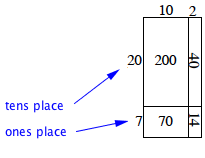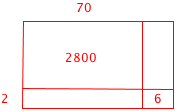### Home > MC1 > Chapter 3 > Lesson 3.3.2 > Problem3-73

3-73.
1.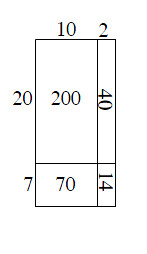3-73. Draw a generic rectangle to help you calculate each product. Homework Help ✎

For each part, write: (total dimension)(total dimension) = sum of individual area parts = total area. For example, the diagram at right shows that (12)(27) = 200 + 40 + 70 +14 = 324.

1. (73)(42)

2. 125 · 81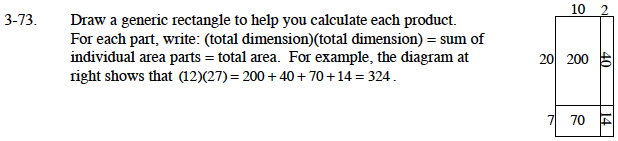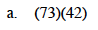Think back to the way you solved the generic rectangles in class.